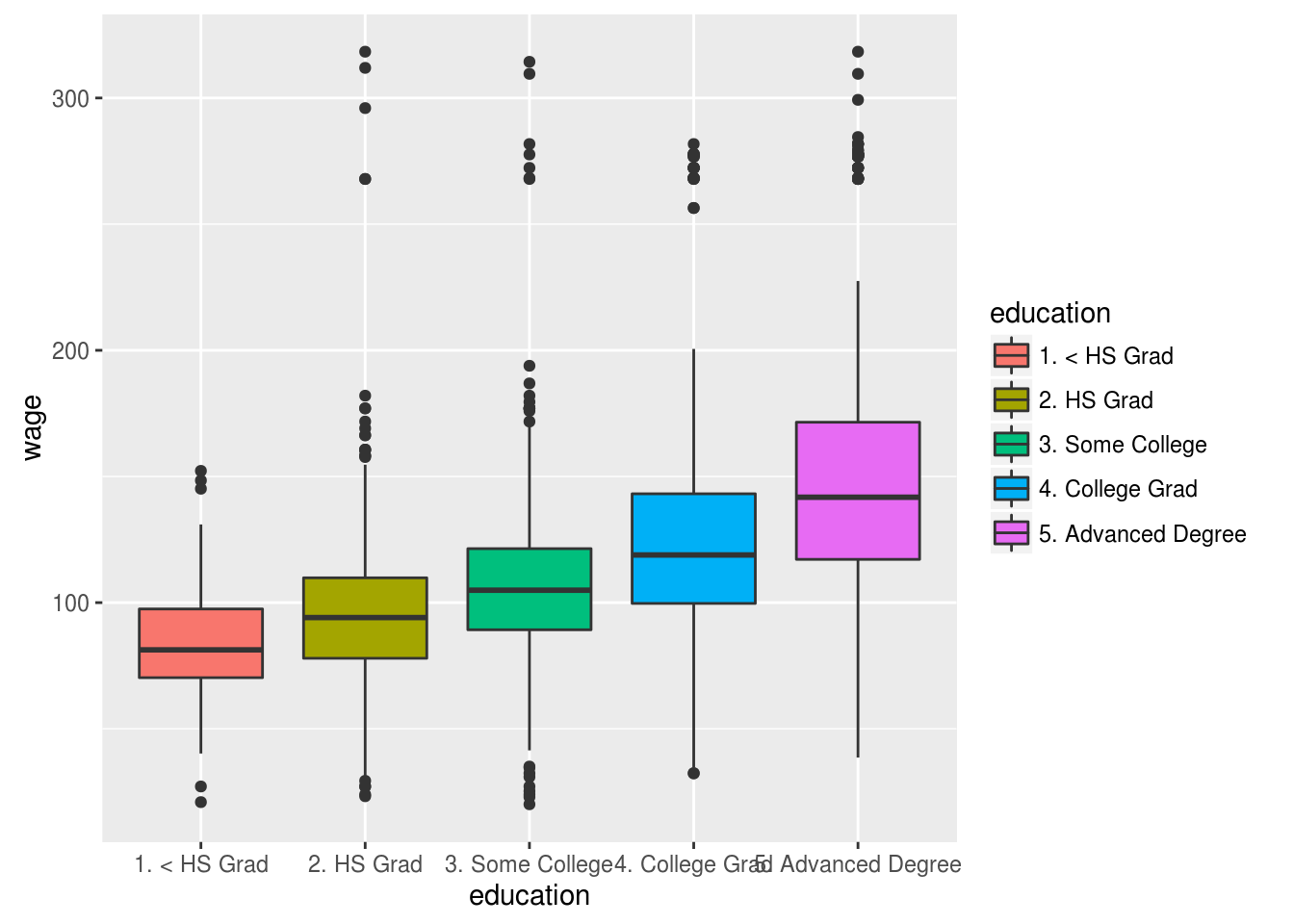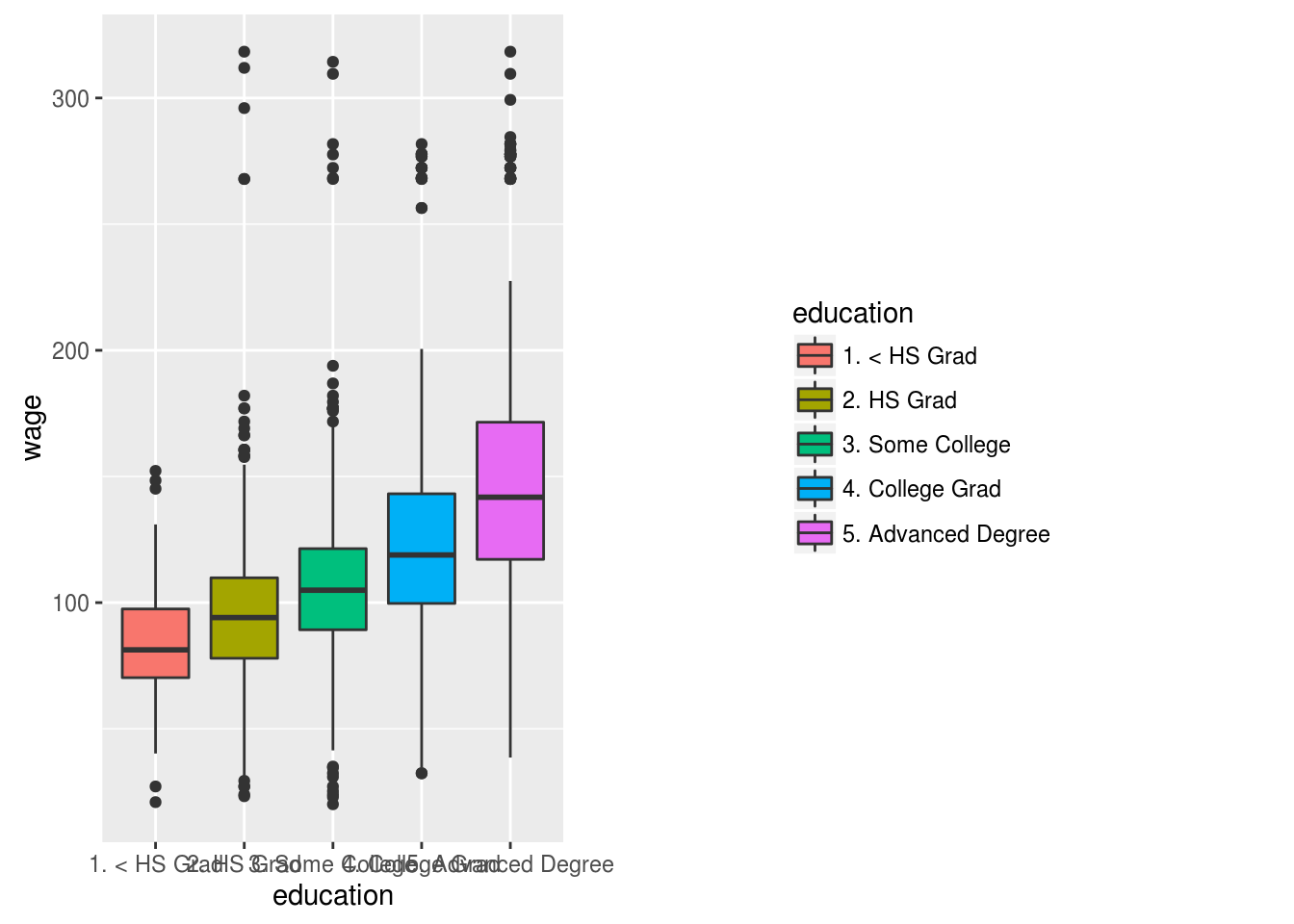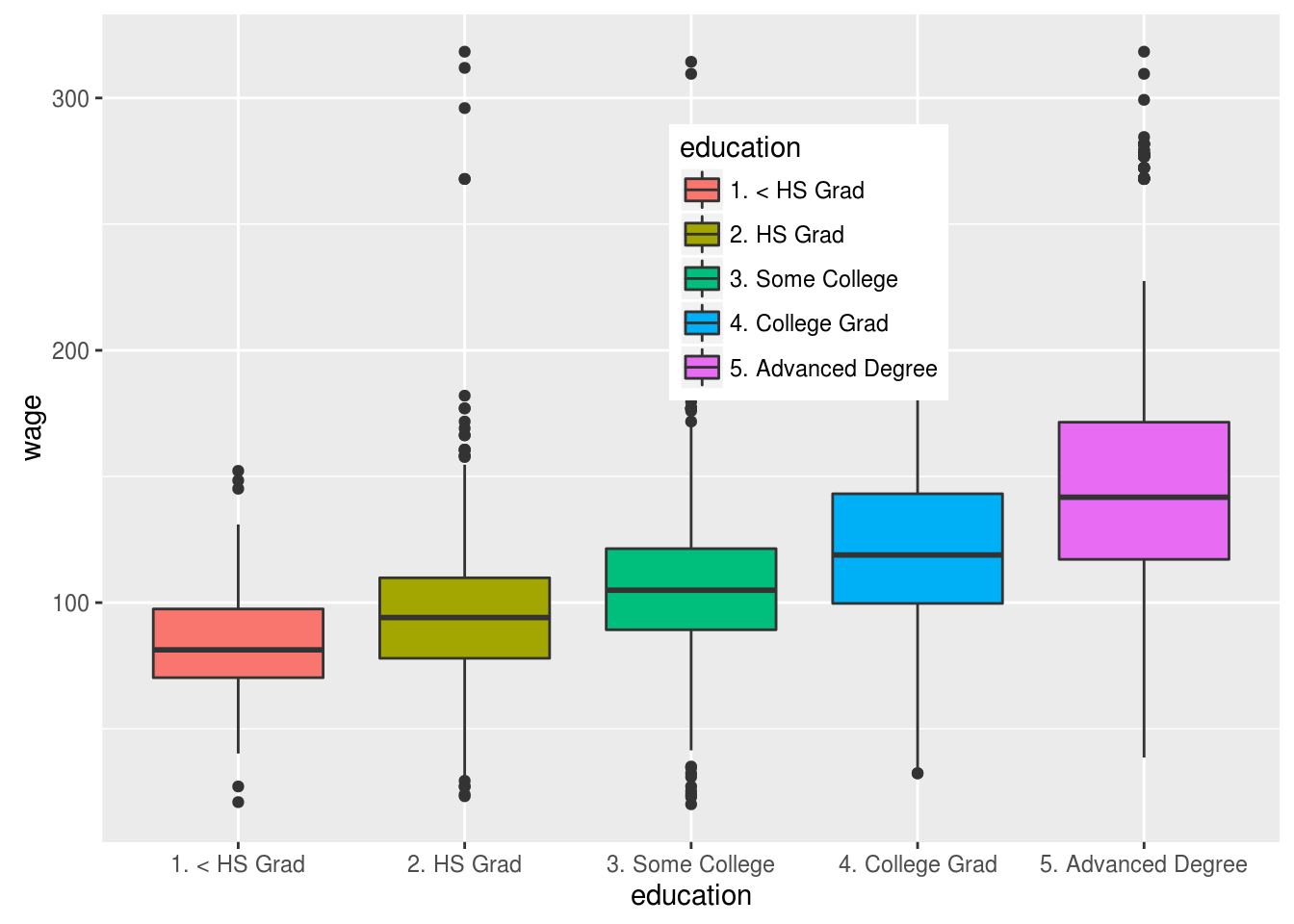# Modifying Legends in ggplot2

This post will provide information on fine tuning the legend of a graph using ggplot2. We will be using the “Wage” dataset from the “ISLR” package. Below is some initial code that is needed to complete the examples. The initial plot is saved as a variable to save time and avoid repeating the same code.

``````library(ggplot2);library(ISLR); library(grid)
myBoxplot<-ggplot(Wage, aes(education, wage,fill=education))+geom_boxplot()
myBoxplot``````The default ggplot has a grey background with grey text. By adding the “theme_bw” function to a plot you can create a plot that has a white background with black text. The code is below.

``myBoxplot+theme_bw()``If you desire, you can also add a rectangle around the legend with the “legend.baclground” argument You can even specify the color of the rectangle as shown below.

``myBoxplot+theme(legend.background=element_rect(color="blue"))``It is also possible to add a highlighting color to the keys in the legend. In the code below we highlight the keys with the color red using the “legend.key” argument

``myBoxplot+theme(legend.key=element_rect(fill="red"))``The code below provides an example of how to change the size of a plot.

``myBoxplot+theme(legend.margin= unit(2, "cm"))``This example demonstrate how to modify the text in a legend. This requires the use of the “legend.text”, along with several other arguments and functions. The code below does the following.

• Size 15 font
• Dark red font color
• Text at 35 degree angle
• Italic font
``myBoxplot + theme(legend.text = element_text(size = 15,color="dark red",angle= 35, face="italic"))``Lastly, you can even move the legend around the plot. The first example moves the legend to the top of the plot using “legend.position” argument. The second example moves the legend based on numerical input. The first number moves the plot from left to right or from 0 being left to 1 being all the way to the right. The second number moves the text from bottom to top with 0 being the bottom and 1 being the top.

``myBoxplot+theme(legend.position="top")````myBoxplot+theme(legend.position=c(.6,.7))``Conclusion

The examples provided here show how much control over plots is possible when using ggplot2. In many ways this is just an introduction into the nuance controlled that is available